﻿ 基于粒子群算法的风电系统调峰策略研究 Study on Peak-Load Control Strategy for Wind Power System Based on Particle Swarm Algorithm

Smart Grid
Vol.07 No.05(2017), Article ID:22251,9 pages
10.12677/SG.2017.75037

Study on Peak-Load Control Strategy for Wind Power System Based on Particle Swarm Algorithm

Xun Mao1, Junli Xia2, Xuchang Zhang1, Guoqiang Zheng1, Yaqiao Luo1, Xiaotao Peng3

1Anhui Electric Power Research Institute, State Grid, Hefei Anhui

2Anhui Hefei Power Supply Company, State Grid, Hefei Anhui

3School of Electrical Engineering, Wuhan University, Wuhan HubeiReceived: Sep. 16th, 2017; accepted: Sep. 28th, 2017; published: Oct. 9th, 2017ABSTRACT

The characteristic of peak-load regulation influenced by random vibration of wind power is different from the power system without the integration of wind power. So exploring peak-load regulation method plays a very important role for keeping power grid integrated with wind power operate in security and economy. In this paper, the demand variety of peak-load regulation led by wind power integration is discussed in the first. Then, taking the aim at consuming wind power as maximum as possible, and taking the minimal generation cost of thermal power, hydropower and pumped storage which are used to regulate the peak-load as optimal object function, the optimal model for peak-load control is established. At the same time, the solution method for the optimal model is developed based on particle swarm algorithm. Finally, the proposed optimal model is used to simulate the peak-load control strategy for a region wind power system. Not only the cooperative peak-load control strategy based on thermal power, hydropower power and pumped storage is arrived in, but also the rationality and feasibility of the optimal model is validated as well.

Keywords:Wind Power System, Peak-Load Control, Particle Swarm Algorithm, Optimal Model

1国网安徽省电力公司电力科学研究院，安徽 合肥

2国网安徽省电力公司合肥供电公司，安徽 合肥

3武汉大学电气工程学院，湖北 武汉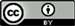1. 引言

2. 风电系统的调峰特性

${P}_{G\text{-}w}\left(t\right)={P}_{L}\left(t\right)-{P}_{w}\left(t\right)$ (1)

3. 基于粒子群算法的调峰策略优化

3.1. 调峰策略的优化模型

${\alpha }_{i}=\frac{{P}_{Gi\mathrm{max}}-{P}_{Gi\mathrm{min}}}{{P}_{Gi\mathrm{max}}}×100%$ (2)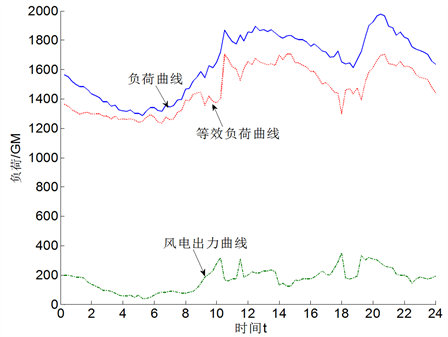Figure 1. Schematic diagram of peak-shavingTable 1. Minimum technical output of thermal generators

$\mathrm{min}F=\underset{t=1}{\overset{T}{\sum }}\underset{i=1}{\overset{{N}_{h}}{\sum }}{C}_{hi}\left(t\right)+\underset{t=1}{\overset{T}{\sum }}\underset{i=1}{\overset{{N}_{c}}{\sum }}\left[\left[{C}_{ci}^{1}\left(t\right)+{C}_{ci}^{2}\left(t\right)\right]+\underset{t=1}{\overset{T}{\sum }}\underset{i=1}{\overset{{N}_{w}}{\sum }}{C}_{si}\left(t\right)$ (3)

s.t.

$\left\{\begin{array}{l}{P}_{gj}+\Delta {P}_{Gi}-{P}_{li}-{U}_{i}\underset{j=1}{\overset{N}{\sum }}{U}_{j}\left({G}_{ij}\mathrm{cos}{\theta }_{ij}+{B}_{ij}\mathrm{sin}{\theta }_{ij}\right)=0\\ {Q}_{gj}-{Q}_{li}-{U}_{i}\underset{j=1}{\overset{N}{\sum }}{U}_{j}\left({G}_{ij}\mathrm{sin}{\theta }_{ij}-{B}_{ij}\mathrm{cos}{\theta }_{ij}\right)=0\\ \underset{k=1}{\overset{{N}_{G}}{\sum }}{\alpha }_{k}{P}_{Gk\mathrm{max}}\ge \underset{m=1}{\overset{{N}_{WG}}{\sum }}\Delta {P}_{mGW\mathrm{max}}+\Delta {P}_{L\mathrm{max}}\\ {P}_{gi\mathrm{min}}\le {P}_{gi}\le {P}_{gi\mathrm{max}},\text{\hspace{0.17em}}\text{\hspace{0.17em}}\text{\hspace{0.17em}}i\in {N}_{g}\\ {U}_{i\mathrm{min}}\le {U}_{i}\le {U}_{i\mathrm{max}},\text{}\text{\hspace{0.17em}}\text{\hspace{0.17em}}\text{\hspace{0.17em}}i\in {N}_{U}\\ {Q}_{gi\mathrm{min}}\le {Q}_{gi}\le {Q}_{gi\mathrm{max}},\text{}i\in {N}_{Q}\end{array}$ (4)

3.2. 基于粒子群算法的求解方法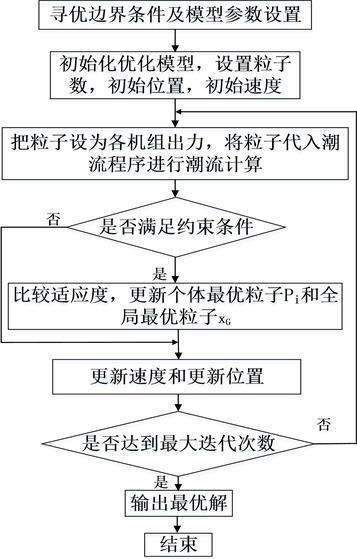Figure 2. Flow chart of the optimal model solution based on particle swarm algorithm

$\left\{\begin{array}{l}{v}_{i}\left(n+1\right)=\omega ×{v}_{i}\left(n\right)+{c}_{1}×rand\left(1\right)×\left({P}_{i}-{x}_{i}\left(n\right)\right)\\ \text{\hspace{0.17em}}\text{\hspace{0.17em}}\text{\hspace{0.17em}}\text{\hspace{0.17em}}\text{\hspace{0.17em}}\text{\hspace{0.17em}}\text{\hspace{0.17em}}\text{\hspace{0.17em}}\text{\hspace{0.17em}}\text{\hspace{0.17em}}\text{\hspace{0.17em}}\text{\hspace{0.17em}}\text{\hspace{0.17em}}\text{\hspace{0.17em}}\text{\hspace{0.17em}}\text{\hspace{0.17em}}+{c}_{2}×rand\left(1\right)×\left({x}_{G}-{x}_{i}\left(n\right)\right)\\ {x}_{i}\left(n+1\right)={x}_{i}\left(n\right)+{v}_{i}\left(n+1\right)\end{array}$ (5)

4. 仿真研究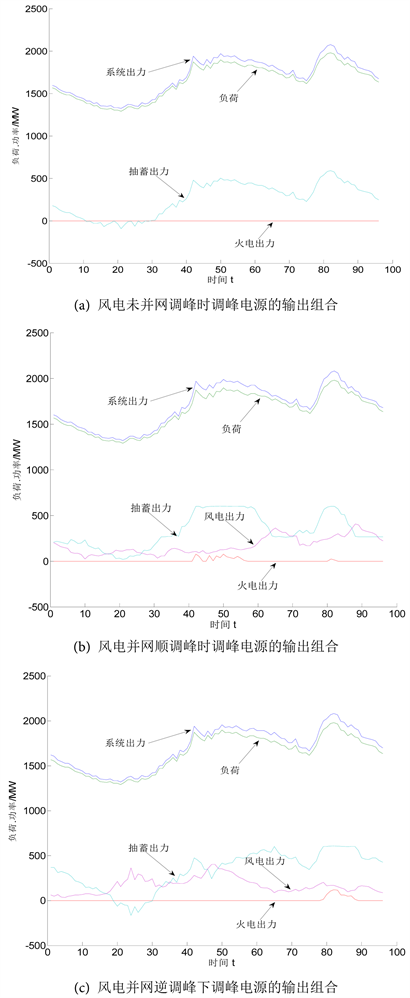Figure 3. Combination of power output used to control peak-load with or without wind power integration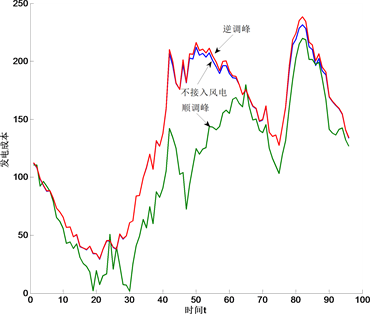Figure 4. Cost comparison of power output used to control peak-load with or without the integration of wind power

5. 结论

Study on Peak-Load Control Strategy for Wind Power System Based on Particle Swarm Algorithm[J]. 智能电网, 2017, 07(05): 332-340. http://dx.doi.org/10.12677/SG.2017.75037

1. 1. 陈炳森, 胡华丽. 我国风电发展概况及展望[J]. 电网技术, 2008, 32(2): 272-275.

2. 2. John, K. and Kanellos, F.D. (2010) Impacts of Large-Scale Wind Penetration on Designing and Operation of Electric Power Systems. IEEE Transactions on Sustainable Energy, 1, 107-115. https://doi.org/10.1109/TSTE.2010.2050348

3. 3. 肖创英, 汪宁渤, 丁坤, 等. 甘肃酒泉风电功率调节方式的研究[J]. 中国电机工程学报, 2010, 30(10): 1-7.

4. 4. 衣立冬, 朱敏奕, 魏磊, 等. 风电并网后西北电网调峰能力的计算方法[J]. 电网技术, 2010, 34(2): 129-132.

5. 5. 李付强, 王彬, 涂少良, 等. 京津唐电网风力发电并网调峰特性分析[J]. 电网技术, 2009, 33(18): 128-132.

6. 6. 张宏宇, 印永华, 申洪, 等. 大规模风电接入后的系统调峰充裕性评估[J]. 中国电机工程学报, 2011, 31(22): 26-31.

7. 7. 张宏宇, 印永华, 申洪, 等. 基于序贯蒙特卡洛方法的风电并网系统调峰裕度评估[J]. 电力系统自动化, 2012, 36(1): 32-37.

8. 8. 刘德伟, 黄越辉, 王伟胜, 等. 考虑调峰和电网输送约束的省级系统风电消纳能力分析[J]. 电力系统自动化, 2011, 352(2): 77-81.

9. 9. Zhang, L.Z. and Cao, F. (2012) Algorithm on Optimal Wind Power Capacity Using Peak Load Regulation Restraints. 2012 IEEE International Conference on Power System Technology (POWERCON), Auckland, IEEE, 1-5.

10. 10. 吴雄, 王秀丽, 李骏, 等. 考虑风电外送的省级系统调峰分析模型[J]. 电网技术, 2013, 37(6): 1578-1583.

11. 11. 孙涛, 许梁, 徐箭, 等. 风电经特高压线路并网时湖北电网调峰方案[J]. 电网技术, 2014, 38(10): 2721-2728.

12. 12. 张宁, 周天睿, 段长刚, 等. 大规模风电接入对电力系统调峰的影响[J]. 电网技术, 2010, 34(1): 152-158.

13. 13. 张志峰, 徐箭, 闫秉科, 等. 考虑特高压送风电的湖北电网调峰方案[J]. 电力自动化设备, 2013, 33(4): 6-11.

14. 14. 黄平. 改进的粒子群算法在电力系统中的应用[D]: [博士学位论文]. 广州: 华南理工大学, 2012.

15. 15. 张庭场, 耿光飞. 基于粒子群算法的中压配电网无功优化[J]. 电网技术, 2012, 36(2): 158-162.